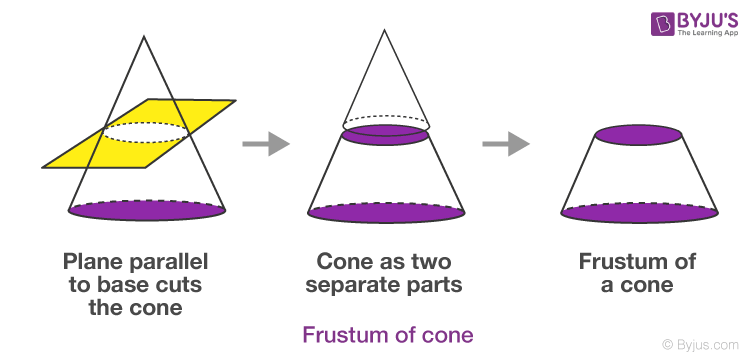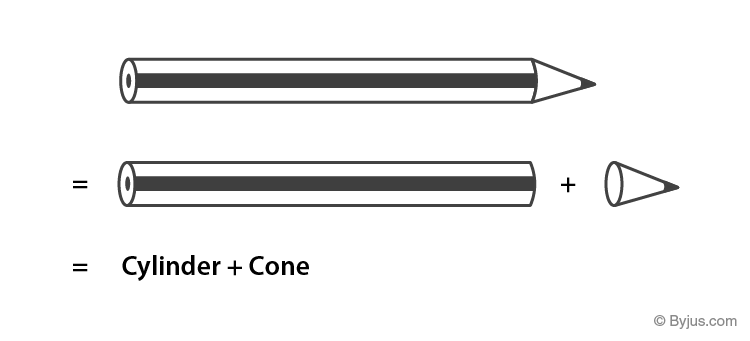# Class 10 Maths Chapter 13 Surface Areas and Volumes MCQs

Class 10 Maths Chapter 13 MCQs (Surface areas and volumes) are provided here online, along with their solutions. Students who are preparing for the board exams can refer to these multiple-choice questions to score good marks in the final examination. These objective questions are framed as per the CBSE syllabus (2022-2023) and NCERT curriculum. To get all class 10 Maths chapter-wise MCQs, Click here.

Students can also get access to Surface Areas and Volumes for Class 10 Notes here.

## Class 10 Maths MCQs for Surface Areas and Volumes

1. The shape of an ice-cream cone is a combination of:

(a) Sphere + cylinder

(b) Sphere + cone

(c) Hemisphere + cylinder

(d) Hemisphere + cone

The shape of an ice-cream cone is a combination of a hemisphere and a cone.

2. If a cone is cut parallel to the base of it by a plane in two parts, then the shape of the top of the cone will be a:

(a) Sphere

(b) Cube

(c) Cone itself

(d) Cylinder

Explanation: If we cut a cone into two parts parallel to the base, then the shape of the upper part remains the same.

3. If we cut a cone in two parts by a plane parallel to the base, then the bottom part left over is the:

(a) Cone

(b) Frustum of cone

(c) Sphere

(d) Cylinder

Explanation: See the figure below4. If r is the radius of the sphere, then the surface area of the sphere is given by;

(a) 4 π r2

(b) 2 π r2

(c) π r2

(d) 4/3 π r2

If r is the radius of the sphere, then the surface area of the sphere is given by 4 π r2.

5. If we change the shape of an object from a sphere to a cylinder, then the volume of cylinder will

(a) Increase

(b) Decrease

(c) Remains unchanged

(d) Doubles

Explanation: If we change the shape of a three-dimensional object, the volume of the new shape will be same.

6. Fifteen solid spheres are made by melting a solid metallic cone of base diameter 2cm and height 15cm. The radius of each sphere is:

(a) ½

(b) ¼

(c) 1/3√2

(d) 1/3√4

Explanation: Volume of 15 spheres = Volume of a cone

15 x (4/3) π r= ⅓ πr2h

5×4 π r= ⅓ π 12(15)

20r= 5

r= 5/20 = ¼

r = 1/3√4

7. The radius of the top and bottom of a bucket of slant height 35 cm are 25 cm and 8 cm. The curved surface of the bucket is:

(a) 4000 sq.cm

(b) 3500 sq.cm

(c) 3630 sq.cm

(d) 3750 sq.cm

Explanation: Curved surface of bucket = π(R+ R2) x slant height (l)

Curved Surface = (22/7) x (25 + 8) x 35

CSA = 22 x 33 x 5 = 3630 sq.cm.

8. If a cylinder is covered by two hemispheres shaped lid of equal shape, then the total curved surface area of the new object will be

(a) 4πrh + 2πr2

(b) 4πrh – 2πr2

(c) 2πrh + 4πr2

(d) 2πrh + 4πr

Explanation: Curved surface area of cylinder = 2πrh

The curved surface area of hemisphere = 2πr2

Here, we have two hemispheres.

So, total curved surface area = 2πrh + 2(2πr2) = 2πrh + 4πr2

9. A tank is made of the shape of a cylinder with a hemispherical depression at one end. The height of the cylinder is 1.45 m and radius is 30 cm. The total surface area of the tank is:

(a) 30 m

(b) 3.3 m

(c) 30.3 m

(d) 3300 m

Explanation: Total surface area of tank = CSA of cylinder + CSA of hemisphere

= 2πrh + 2πr2= 2π r(h + r)

= 2 x 22/7 x 30(145 + 30) cm2

=33000 cm2

= 3.3 m2

10. If we join two hemispheres of same radius along their bases, then we get a;

(a) Cone

(b) Cylinder

(c) Sphere

(d) Cuboid

If we join two hemispheres of same radius along their bases, then we get a Sphere.

11. A cylindrical pencil sharpened at one edge is the combination of

(a) a cone and a cylinder

(b) frustum of a cone and a cylinder

(c) a hemisphere and a cylinder

(d) two cylinders

Answer: (a) a cone and a cylinder

A cylindrical pencil sharpened at one edge is the combination of a cone and a cylinder.12. A shuttle cock used for playing badminton has the shape of the combination of

(a) a cylinder and a sphere

(b) a cylinder and a hemisphere

(c) a sphere and a cone

(d) frustum of a cone and a hemisphere

Answer: (d) frustum of a cone and a hemisphere

A shuttle cock used for playing badminton has the shape of the combination of frustum of a cone and a hemisphere.

13. A hollow cube of internal edge 22 cm is filled with spherical marbles of diameter 0.5 cm and it is assumed that 1/8 space of the cube remains unfilled. Then the number of marbles that the cube can accommodate is

(a) 142296

(b) 142396

(c) 142496

(d) 142596

Explanation:

Volume of cube = 223 = 10648 cm3

Volume of cube that remains unfilled = (1/8) × 10648 = 1331 cm3

volume occupied by spherical marbles = 10648 − 1331 = 9317 cm3

Radius of the spherical marble = 0.5/2 = 0.25 cm = 1/4 cm

Volume of 1 spherical marble = (4/3) × (22/7) × (1/4)3 = 11/168 cm3

Numbers of spherical marbles = n = 9317 × (11/168) = 142296

14. A solid piece of iron in the form of a cuboid of dimensions 49 cm × 33 cm × 24 cm, is moulded to form a solid sphere. The radius of the sphere is

(a) 21 cm

(b) 23 cm

(c) 25 cm

(d) 19 cm

Explanation:

For the given cuboid,

Length, l = 49 cm

Height, h = 24 cm

Volume of cube = 49 × 33 × 24 cm3

Let r be the radius of the sphere.

Volume of sphere = 4/3 πr3

Volume of cuboid = volume of sphere molded

49 × 33 × 24 = 4/3 πr3

⇒ πr3 = 29106

⇒ r3 = 29106 × (22/7)

⇒ r3 = 9261

⇒ r3 = (21)3

⇒ r = 21 cm

Hence, the radius of sphere is 21 cm

15. A right circular cylinder of radius r cm and height h cm (h>2r) just encloses a sphere of diameter

(a) r cm

(b) 2r cm

(c) h cm

(d) 2h cm

Explanation:

Height = h > 2r

Since the sphere is enclosed by a cylinder, the radius of the cylinder will be the radius of the sphere.

Therefore, diameter of sphere = 2r cm

16. The diameters of the two circular ends of the bucket are 44 cm and 24 cm. The height of the bucket is 35 cm. The capacity of the bucket is

(a) 32.7 litres

(b) 33.7 litres

(c) 34.7 litres

(d) 31.7 litres

Explanation:

Given,

The height of the bucket = h = 35 cm

Diameter of one circular end of bucket = 44 cm

Then the radius R = 22 cm

Diameter of another end = 24 cm

Then the radius r = 12 cm

We know that Volume of the bucket = (1/3)πh[R2 + r2 + Rr]

= (1/3) × (22/7) × 35 × [(22)2 + (12)2 + 22 × 12]

= (35/3) × (22/7) × (484 + 144 + 264)

= (5 × 22 × 892)/3

= 32706.6 cm3

= 32.7 litres.

17. Two identical solid cubes of side a are joined end to end. Then the total surface area of the resulting cuboid is

(a) 12a2

(b) 10a2

(c) 8a2

(d) 11a2

Explanation:

The total surface area of a cube having side a = 6a2

If two identical faces of side a are joined together, then the total surface area of the cuboid so formed is 10a2.

18. A solid cylinder of radius r and height h is placed over another cylinder of same height and radius. The total surface area of the shape so formed is

(a) 4πrh + 4πr2

(b) 2πrh + 4πr2

(c) 2πrh + 2πr2

(d) 4πrh + 2πr2

Explanation:

We know that,

The total surface area of cylinder = 2πrh + 2πr2

When one cylinder is placed over the other cylinder of same height and radius, then height of the new cylinder will be 2h and radius will be r.

Thus, the total surface area of the shape so formed = 2πr(2h) + 2πr2 = 4πrh + 2πr2

19. The number of shots each having diameter 3 cm can be made from a cuboidal lead solid of dimensions 9 cm × 11 cm × 12 cm is approximately equal to

(a) 84

(b) 90

(c) 92

(d) 80

Explanation:

Volume of cuboidal lead solid = 9 cm × 11 cm × 12 cm = 1188 cm3

Volume of each shot = (4/3)πr3

= (4/3) × (22/7) × 1.5 × 1.5 × 1.5

= 14.143 cm3

Number of lead shots can be made = 1188/14.143 = 84 (approx.)

20. Two identical solid hemispheres of equal base radius r cm are stuck together along their bases. The total surface area of the combination is

(a) 6πr2

(b) 5πr2

(c) 4πr2

(d) 3πr2